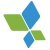0+0+0+0+0+0+0+0+0+0+0+0+0+0+0+0+0+0+0+0+0+In this paper, we propose a method for semantic segmentation of pedestrian trajectories based on pedestrian behavior models, or agents. The agents model the dynamics of pedestrian movements in two-dimensional space using a linear dynamics model and common start and goal locations of trajectories. First, agent models are estimated from the trajectories obtained from image sequences. Our method is built on top of the Mixture model of Dynamic pedestrian Agents (MDA); however, the MDA's trajectory modeling and estimation are improved. Then, the trajectories are divided into semantically meaningful segments. The subsegments of a trajectory are modeled by applying a hidden Markov model using the estimated agent models. Experimental results with a real trajectory dataset show the effectiveness of the proposed method as compared to the well-known classical Ramer-Douglas-Peucker algorithm and also to the original MDA model.0+0+0+下载预览0+0+0+0+0+0+0+0+0+0+0+0+0+0+0+0+0+0+0+0+0+
Top